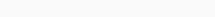# Math Puzzles Puzzle 36 Answer AndroidMath puzzle game level 36 solution with detailed hints and explanation, Scroll below to find out.

Math puzzles increase your logical thinking. The objective of the game is to find patterns hidden in the puzzle in order to arrive at the solution. Challenge yourself with different levels of math puzzles and stretch the limits of your intelligence.

### Math Puzzles Puzzle 36:

if, 2,4=16

3,5=45

4,6=96

5,7=175

then 6,8=?Solution/Hint: A*A*B

6*6*8=288

If there is any Doubt or you are unable to understand the solution, then please let me know in comments, I will surely help you.

### One thought on “Math Puzzles Game Level 36 Answer with Solution”

•June 15, 2021 at 21:34